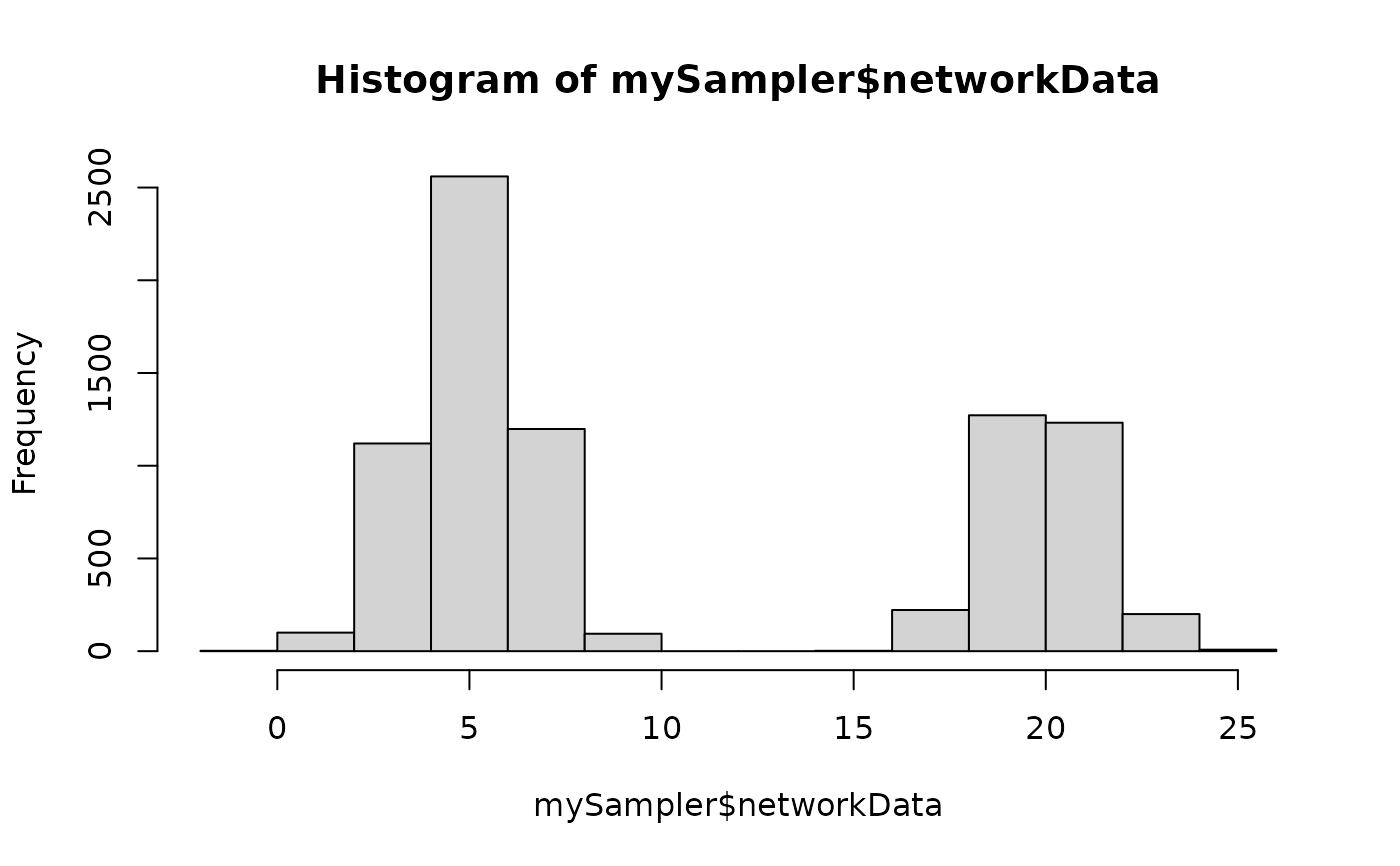This function samples a simple Stochastic Block Models, with various model for the distribution of the edges: Bernoulli, Poisson, or Gaussian models, and possibly with covariates

sampleSimpleSBM(
nbNodes,
blockProp,
connectParam,
model = "bernoulli",
directed = FALSE,
dimLabels = c(node = "nodeName"),
covariates = list(),
covariatesParam = numeric(0)
)

## Arguments

nbNodes number of nodes in the network parameters for block proportions list of parameters for connectivity with a matrix of means 'mean' and an optional matrix of variances 'var', the sizes of which must match blockProp length character describing the model for the relation between nodes ('bernoulli', 'poisson', 'gaussian', ...). Default is 'bernoulli'. logical, directed network or not. Default is FALSE. an optional list of labels for each dimension (in row, in column) a list of matrices with same dimension as mat describing covariates at the edge level. No covariate per Default. optional vector of covariates effect. A zero length numeric vector by default.

## Value

an object with class SimpleSBM

## Examples

### =======================================
### SIMPLE BINARY SBM (Bernoulli model)
## Graph parameters
nbNodes  <- 90
blockProp <- c(.5, .25, .25) # group proportions
means <- diag(.4, 3) + 0.05  # connectivity matrix: affiliation network
# In Bernoulli SBM, parameters is a list with a
# matrix of means 'mean' which are probabilities of connection
connectParam <- list(mean = means)

## Graph Sampling
mySampler <- sampleSimpleSBM(nbNodes, blockProp, connectParam, model = 'bernoulli')
plot(mySampler)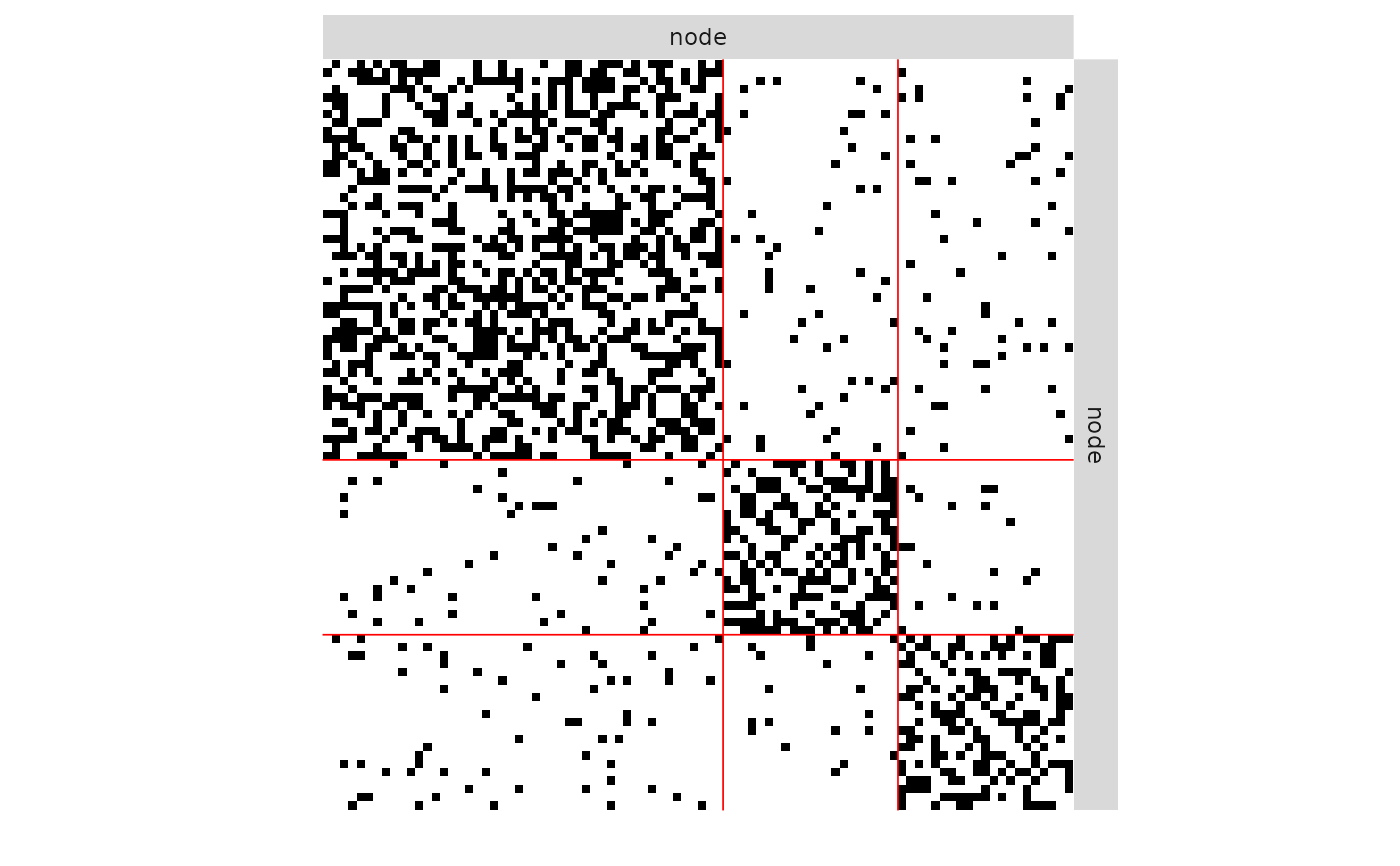plot(mySampler)plot(mySampler,type='meso')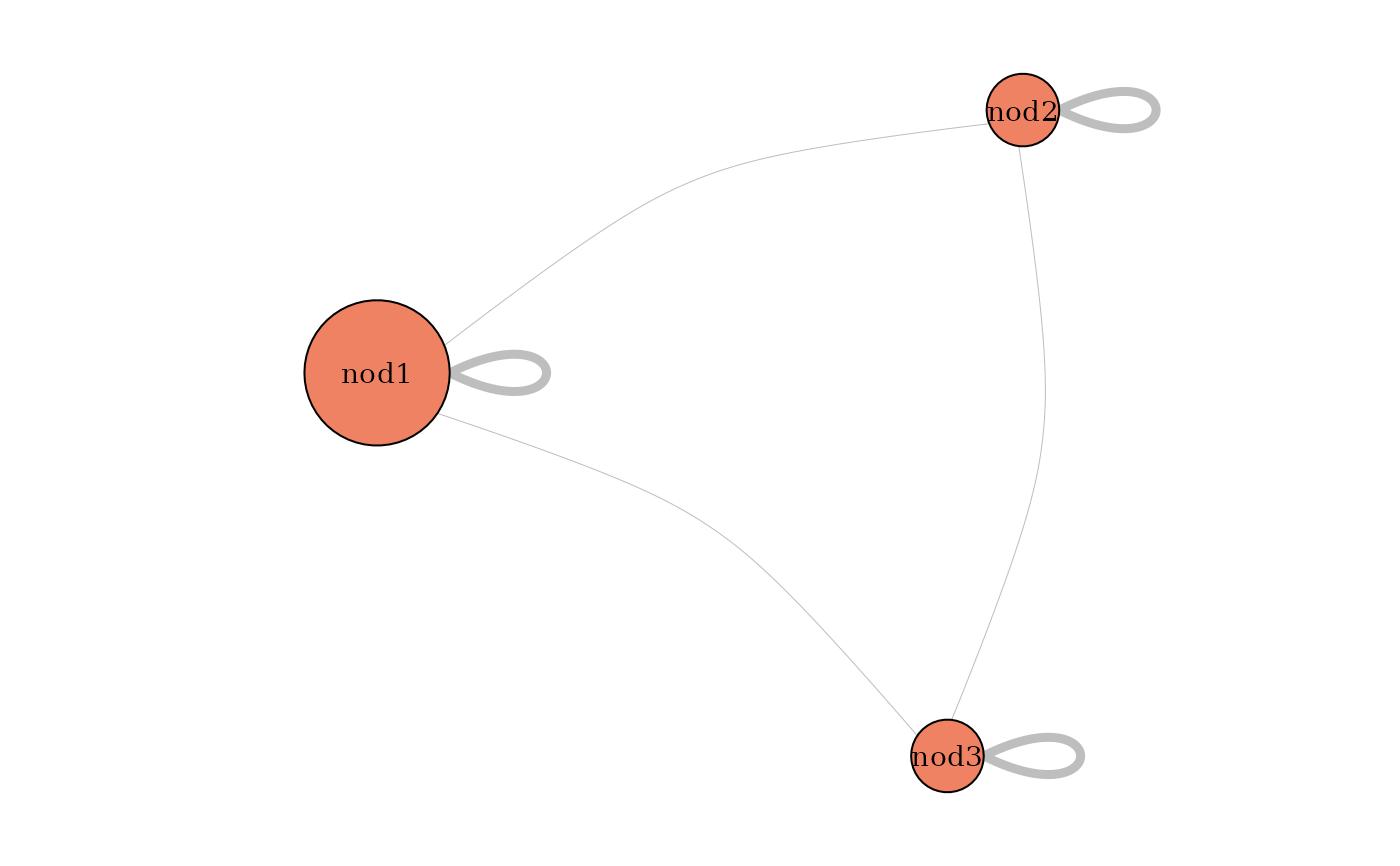hist(mySampler$networkData)### ======================================= ### SIMPLE POISSON SBM ## Graph parameters nbNodes <- 90 blockProp <- c(.5, .25, .25) # group proportions means <- diag(15., 3) + 5 # connectivity matrix: affiliation network # In Poisson SBM, parameters is a list with # a matrix of means 'mean' which are a mean integer value taken by edges connectParam <- list(mean = means) ## Graph Sampling mySampler <- sampleSimpleSBM(nbNodes, blockProp, list(mean = means), model = "poisson") plot(mySampler)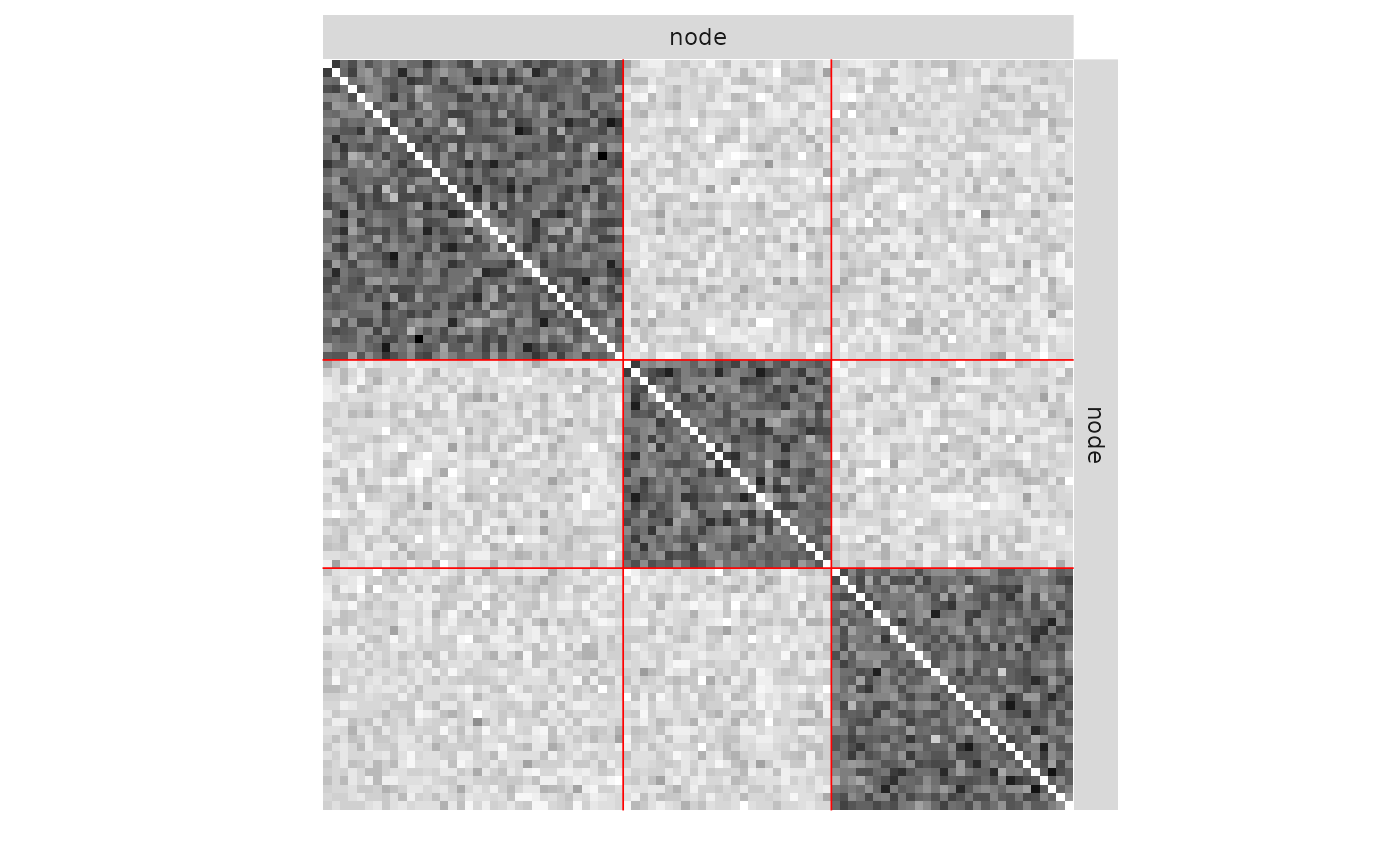plot(mySampler,type='meso')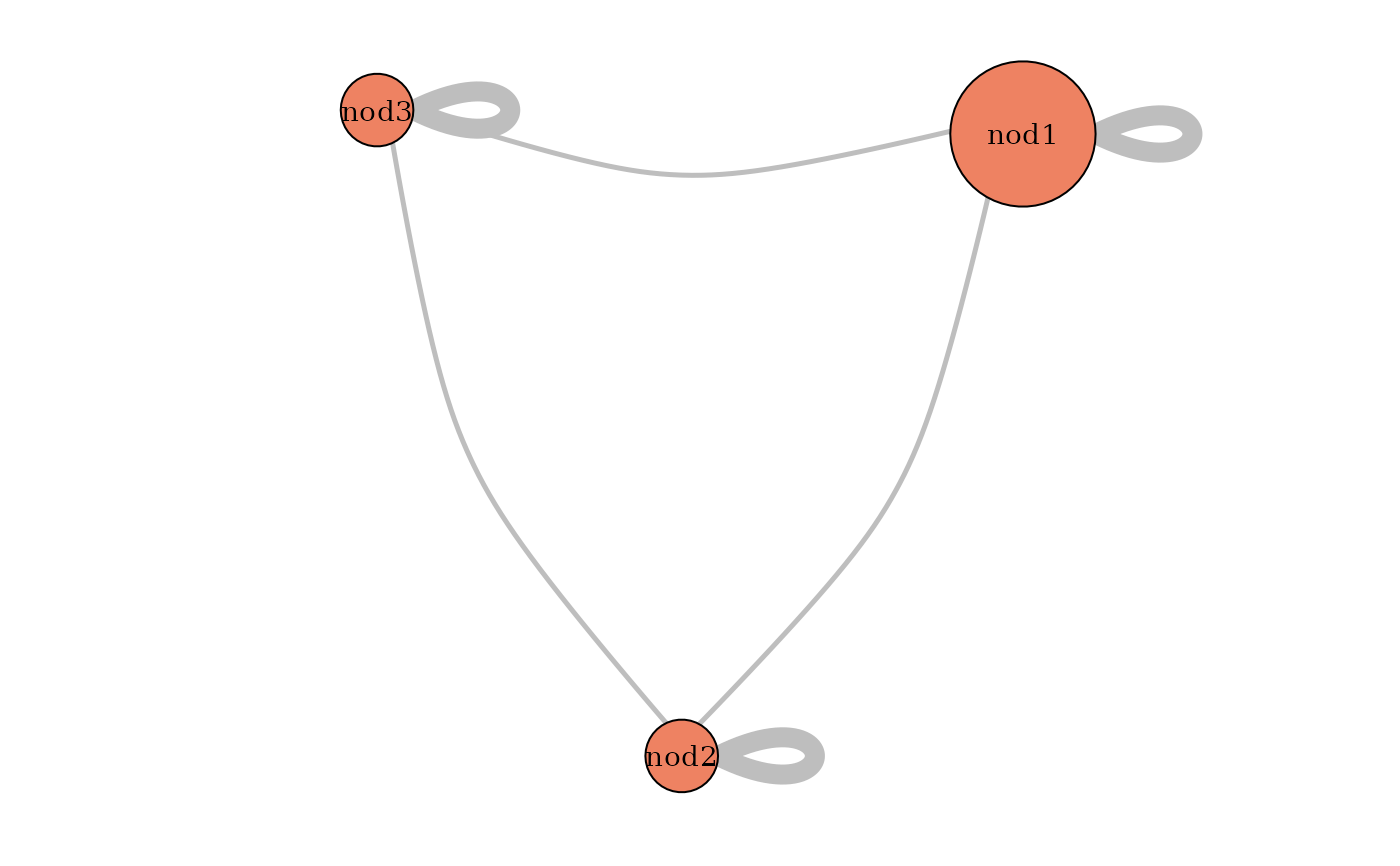hist(mySampler$networkData)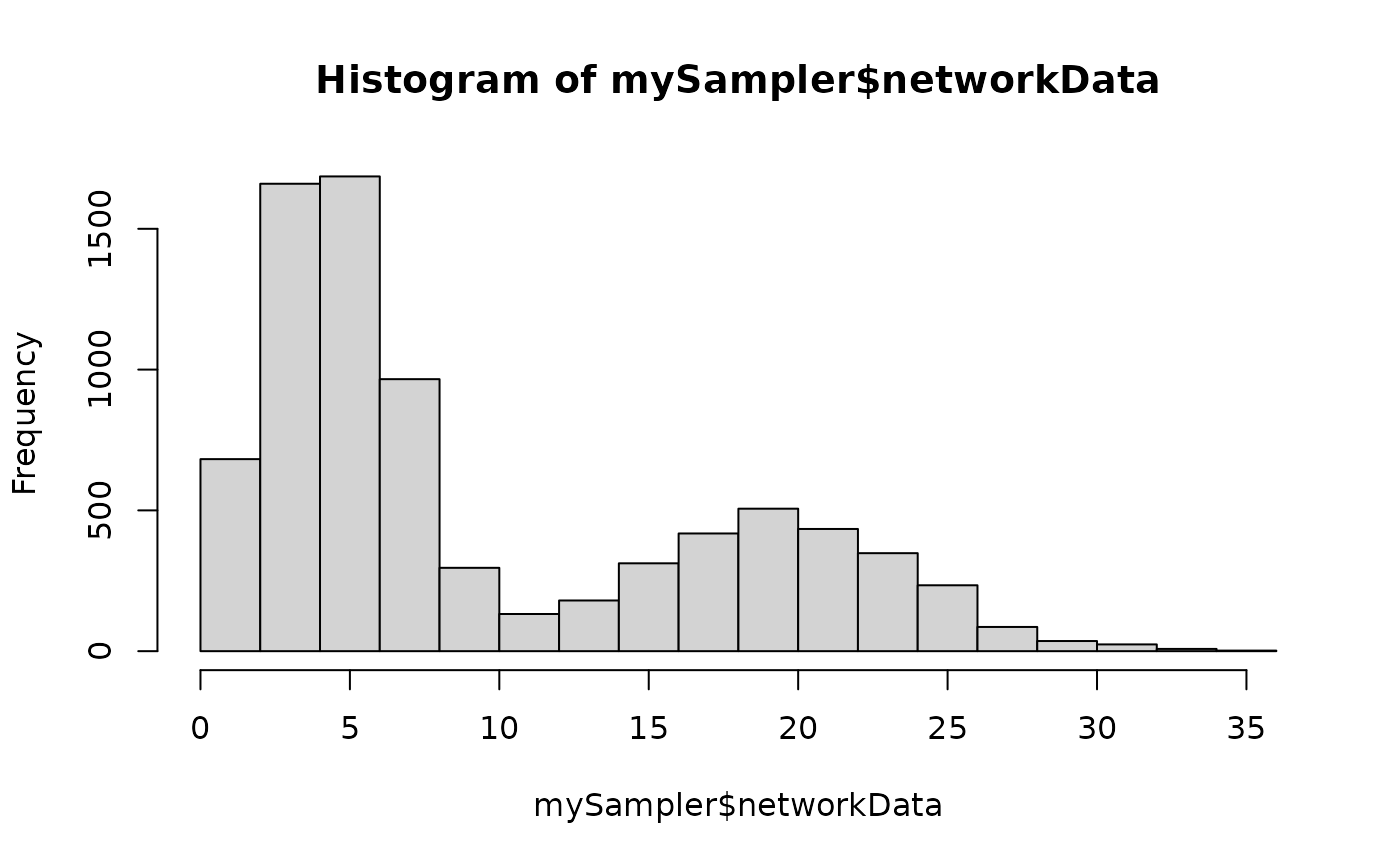### =======================================
### SIMPLE GAUSSIAN SBM
## Graph parameters
nbNodes  <- 90
blockProp <- c(.5, .25, .25)      # group proportions
means <- diag(15., 3) + 5 # connectivity matrix: affiliation network
# In Gaussian SBM, parameters is a list with
# a matrix of means 'mean' and a matrix of variances 'var'
connectParam <- list(mean = means, var = 2)

## Graph Sampling
mySampler <- sampleSimpleSBM(nbNodes, blockProp, connectParam, model = "gaussian")
plot(mySampler)plot(mySampler,type='meso')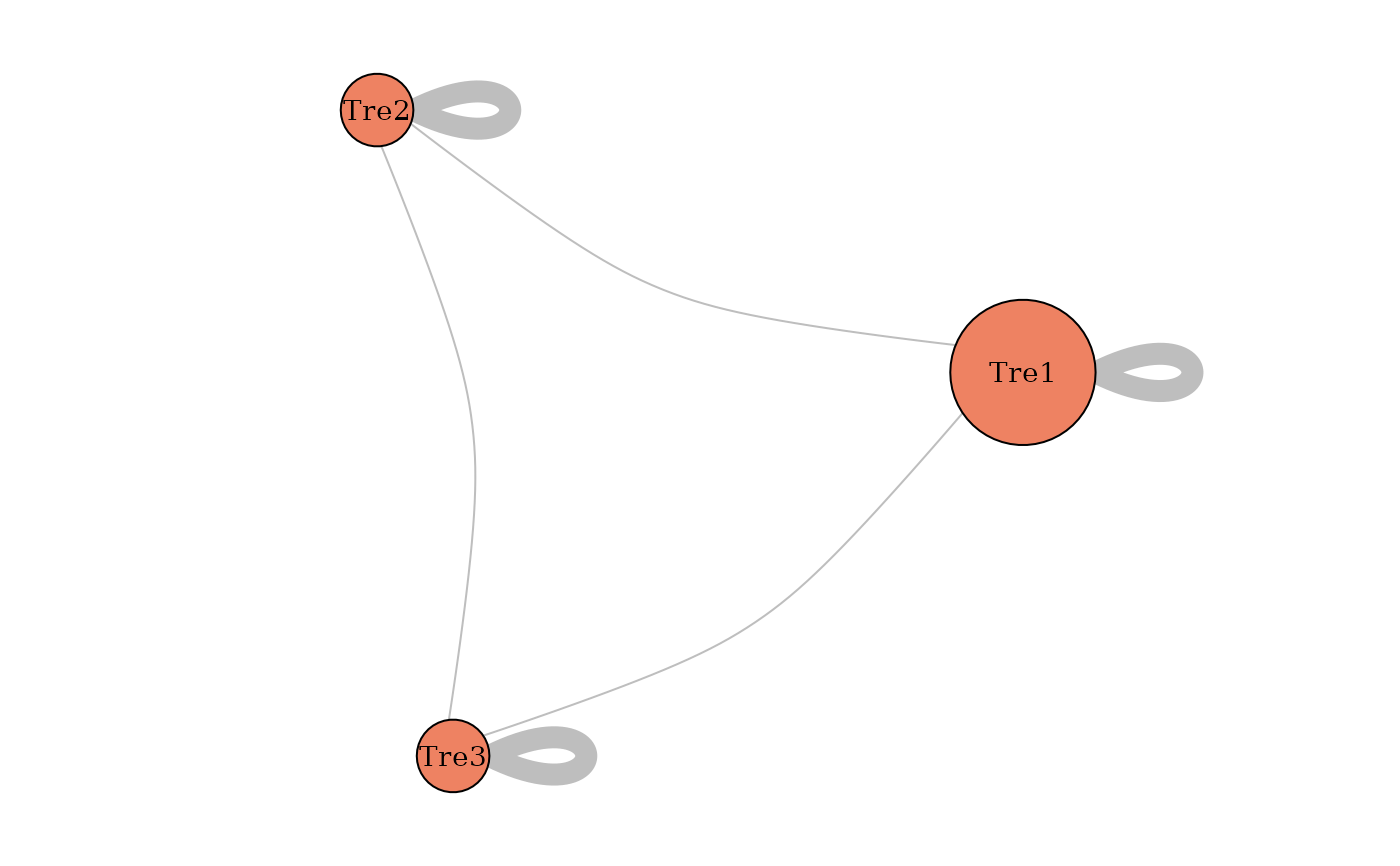hist(mySampler\$networkData)